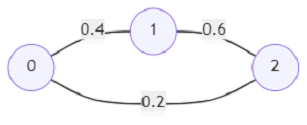# Program to find path with maximum probability using Python

Suppose we have an undirected weighted graph with n nodes (nodes are numbered from 0 onwards), This graph is given as input using edge list, for each edge e, it has a probability of success of traversing that edge probability[e]. We also have start and end nodes, we have to find the path with the maximum probability of success to go from start to end and return its success probability. If we cannot find any path, then return 0.

So, if the input is likethen the output will be 0.24 because there are two paths from node 0 to 2, one with probability 0.2, another one via node 1 has probability 0.4*0.6 = 0.24, this is maximum.

To solve this, we will follow these steps −

• g := make graph from given edge list and use probability value as weight

• q := a queue data structure

• insert (start, 1) into q

• visited := a map to hold visited node

• while q is not empty, do

• (node, prob) := first item of q and delete it from q

• if visited[node] > prob, then

• go for next iteration

• otherwise,

• visited[node] := prob

• for each adjacent node adj and probability nextProb in g[node], do

• if visited[adj] < prob * nextProb, then

• insert (adj, prob * nextProb) at the end of q

• return visited[end]

Let us see the following implementation to get better understanding −

## Example

Live Demo

from collections import defaultdict, deque
def solve(edges, probability, start, end):
g = defaultdict(list)
for i in range(len(edges)):
src, dst = edges[i], edges[i]
prob = probability[i]
g[src].append((dst, prob))
g[dst].append((src, prob))
q = deque()
q.append((start, 1))
visited = defaultdict(int)
while q:
node, prob = q.popleft()
if visited[node] > prob:
continue
else:
visited[node] = prob
if visited[adj] < prob * nextProb:
return visited[end]
edges = [[0,1],[1,2],[0,2]]
probability = [0.5,0.5,0.2]
start = 0
end = 2
print(solve(edges, probability, start, end))

## Input

[[0,1],[1,2],[0,2]], [0.5,0.5,0.2], 0, 2

## Output

0.25

Updated on: 29-May-2021

315 Views